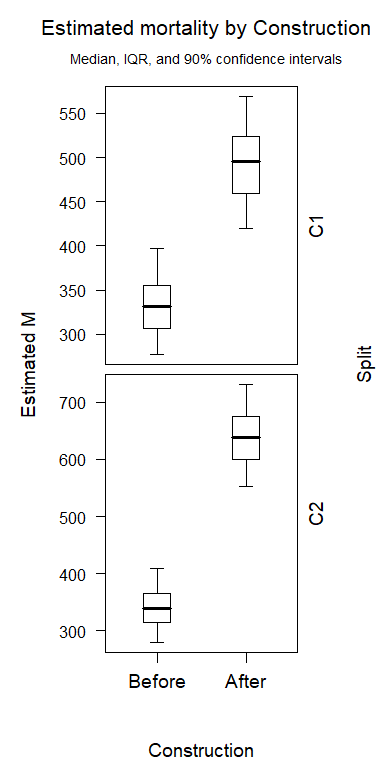# GenEst - 3. Command Line Walkthrough

#### 2021-06-16

This vignette walks through an example of GenEst at the command line and was constructed using GenEst version 1.4.6 on 2021-06-16.

## Data

For this vignette, we will be using a completely generic, mock dataset provided with the GenEst package, which contains Searcher Efficiency (SE), Carcass Persistence (CP), Search Schedule (SS), Density Weighted Proportion (DWP), and Carcass Observation (CO) Data.

data(mock)
names(mock)
#>  "SE"  "CP"  "SS"  "DWP" "CO"

## Searcher Efficiency

### Single Searcher Efficiency Model

The central function for searcher efficiency analyses is pkm, which, in its most basic form, conducts a singular searcher efficiency analysis (i.e., a singular set of $$p$$ and $$k$$ formulae and a singular size classification of carcasses). As a first example, we will ignore the size category and use intercept-only models for both $$p$$ and $$k$$:

data_SE <- mock$SE pkModel <- pkm(formula_p = p ~ 1, formula_k = k ~ 1, data = data_SE) Here, we have taken advantage of pkm’s default behavior of selecting observation columns (see ?pkm for details). head(data_SE) #> seID Visibility HabitatType Season Size Search1 Search2 Search3 Search4 #> 1 se1 L HT1 SF S 1 NA NA NA #> 2 se2 L HT1 SF S 1 NA NA NA #> 3 se3 L HT1 WS S 0 0 0 0 #> 4 se4 L HT1 WS S 1 NA NA NA #> 5 se5 L HT1 WS S 0 1 NA NA #> 6 se6 L HT1 SF S 1 NA NA NA If we wanted to explicitly control the observations, we would use the obsCol argument: pkModel <- pkm(formula_p = p ~ 1, formula_k = k ~ 1, data = data_SE, obsCol = c("Search1", "Search2", "Search3", "Search4") ) Note that the search observations must be entered in order such that no carcasses have non-detected observations (i.e., 0) after detected observations (i.e., 1). Further, no carcasses can be detected more than once. If successfully fit, a pkm model output contains a number of elements, some printed automatically: pkModel #>$call
#> pkm0(formula_p = formula_p, formula_k = formula_k, data = data,
#>     obsCol = obsCol, kFixed = kFixed, kInit = kInit, CL = CL,
#>     quiet = quiet)
#>
#> $formula_p #> p ~ 1 #> #>$formula_k
#> k ~ 1
#>
#> $predictors #> character(0) #> #>$AICc
#>  1145
#>
#> $convergence #>  0 #> #>$cell_pk
#>   cell   n p_median    p_lwr    p_upr k_median   k_lwr    k_upr
#> 1  all 480 0.568447 0.531657 0.604497 0.599059 0.54296 0.652677
#>
#> $CL #>  0.9 and others available upon request: names(pkModel) #>  "call" "data" "data0" "formula_p" "formula_k" #>  "predictors" "predictors_p" "predictors_k" "AIC" "AICc" #>  "convergence" "varbeta" "cellMM_p" "cellMM_k" "nbeta_p" #>  "nbeta_k" "betahat_p" "betahat_k" "cells" "ncell" #>  "cell_pk" "CL" "observations" "carcCells" "loglik" #>  "pOnly" "data_adj" pkModel$cells
#>   group CellNames
#> 1   all       all

The plot function has been defined for pkm objects, such that one can simply run

plot(pkModel)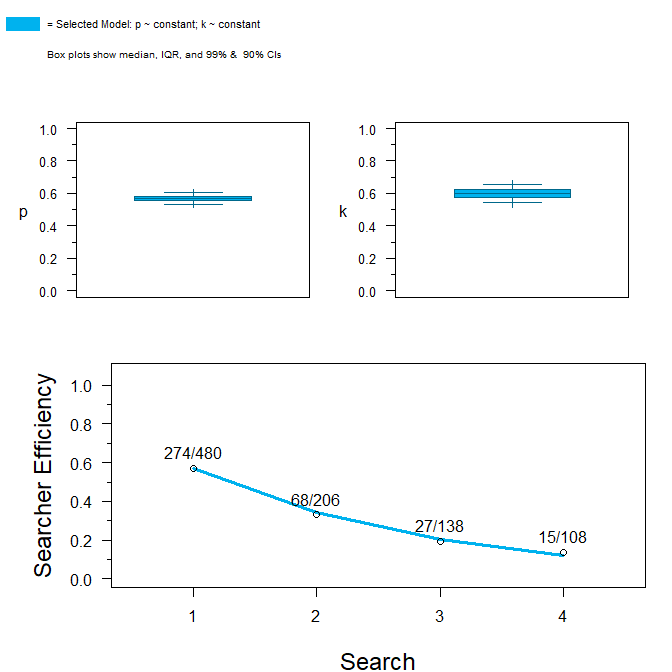to visualize the model’s output.

You can generate random draws of the $$p$$ and $$k$$ parameters for each cell grouping (in pkModel there are no predictors, so there is one cell grouping called “all”) using the rpk function which, like other r* functions in R (e.g., rnorm, runif) takes the number of random draws (n) as the first argument:

rpk(n = 10, pkModel)
#> $all #> p k #> [1,] 0.5581535 0.5809058 #> [2,] 0.5877854 0.5379979 #> [3,] 0.6039170 0.5692513 #> [4,] 0.5471520 0.6189545 #> [5,] 0.5609113 0.5263933 #> [6,] 0.5819523 0.5973587 #> [7,] 0.5749771 0.5909346 #> [8,] 0.5844859 0.5762682 #> [9,] 0.5646682 0.6336280 #> [10,] 0.5529392 0.6245416 You can complicate the $$p$$ and $$k$$ formulae independently pkm(formula_p = p ~ Visibility, formula_k = k ~ HabitatType, data = data_SE, obsCol = c("Search1", "Search2", "Search3", "Search4") ) #>$call
#> pkm0(formula_p = formula_p, formula_k = formula_k, data = data,
#>     obsCol = obsCol, kFixed = kFixed, kInit = kInit, CL = CL,
#>     quiet = quiet)
#>
#> $formula_p #> p ~ Visibility #> #>$formula_k
#> k ~ HabitatType
#>
#> $predictors #>  "Visibility" "HabitatType" #> #>$AICc
#>  1149.67
#>
#> $convergence #>  0 #> #>$cell_pk
#>    cell  n p_median    p_lwr    p_upr k_median    k_lwr    k_upr
#> 1 H.HT1 80 0.564028 0.503246 0.622945 0.560177 0.481763 0.635699
#> 2 L.HT1 80 0.577879 0.516334 0.637098 0.560177 0.481763 0.635699
#> 3 M.HT1 80 0.563479 0.504787 0.620445 0.560177 0.481763 0.635699
#> 4 H.HT2 80 0.564028 0.503246 0.622945 0.631251 0.556647 0.700066
#> 5 L.HT2 80 0.577879 0.516334 0.637098 0.631251 0.556647 0.700066
#> 6 M.HT2 80 0.563479 0.504787 0.620445 0.631251 0.556647 0.700066
#>
#> $CL #>  0.9 And you can fix $$k$$ at a nominal value between 0 and 1 (inclusive) using the kFixed argument pkm(formula_p = p ~ Visibility, kFixed = 0.7, data = data_SE, obsCol = c("Search1", "Search2", "Search3", "Search4")) #>$call
#> pkm0(formula_p = formula_p, formula_k = formula_k, data = data,
#>     obsCol = obsCol, kFixed = kFixed, kInit = kInit, CL = CL,
#>     quiet = quiet)
#>
#> $formula_p #> p ~ Visibility #> #>$formula_k
#> fixedk
#>    0.7
#>
#> $predictors #>  "Visibility" #> #>$AICc
#>  1155.63
#>
#> $convergence #>  0 #> #>$cell_pk
#>   cell   n p_median    p_lwr    p_upr k_median k_lwr k_upr
#> 1    H 160 0.531356 0.474326 0.587579      0.7   0.7   0.7
#> 2    L 160 0.544816 0.487026 0.601424      0.7   0.7   0.7
#> 3    M 160 0.537882 0.482043 0.592786      0.7   0.7   0.7
#>
#> $CL #>  0.9 ### Set of Searcher Efficiency Models If the arg allCombos = TRUE is provided, pkm fits a set of pkm models defined as all allowable models simpler than, and including, the provided model for both formulae (where “allowable” means that any interaction terms have all component terms included in the model). Consider the following model set analysis, where visibility and habitat type are included in the $$p$$ formula but only habitat type is in the $$k$$ formula. This generates a set of 10 models: pkmModSet <- pkm(formula_p = p ~ Visibility*HabitatType, formula_k = k ~ HabitatType, data = data_SE, obsCol = c("Search1", "Search2", "Search3", "Search4"), allCombos = TRUE ) class(pkmModSet) #>  "pkmSet" "list" names(pkmModSet) #>  "p ~ Visibility * HabitatType; k ~ HabitatType" #>  "p ~ Visibility + HabitatType; k ~ HabitatType" #>  "p ~ HabitatType; k ~ HabitatType" #>  "p ~ Visibility; k ~ HabitatType" #>  "p ~ 1; k ~ HabitatType" #>  "p ~ Visibility * HabitatType; k ~ 1" #>  "p ~ Visibility + HabitatType; k ~ 1" #>  "p ~ HabitatType; k ~ 1" #>  "p ~ Visibility; k ~ 1" #>  "p ~ 1; k ~ 1" The plot function is defined for the pkmSet class, and by default, creates a new plot window on command for each sub-model. If we want to only plot a specific single (or subset) of models from the full set, we can utilize the specificModel argument: plot(pkmModSet, specificModel = "p ~ Visibility + HabitatType; k ~ 1") The resulting model outputs can be compared in an AICc table aicc(pkmModSet) #> p Formula k Formula AICc <U+0394>AICc #> 10 p ~ 1 k ~ 1 1145.00 0.00 #> 5 p ~ 1 k ~ HabitatType 1145.70 0.70 #> 3 p ~ HabitatType k ~ HabitatType 1146.57 1.57 #> 8 p ~ HabitatType k ~ 1 1146.76 1.76 #> 9 p ~ Visibility k ~ 1 1148.96 3.96 #> 4 p ~ Visibility k ~ HabitatType 1149.67 4.67 #> 2 p ~ Visibility + HabitatType k ~ HabitatType 1150.55 5.55 #> 7 p ~ Visibility + HabitatType k ~ 1 1150.73 5.73 #> 1 p ~ Visibility * HabitatType k ~ HabitatType 1153.45 8.45 #> 6 p ~ Visibility * HabitatType k ~ 1 1153.49 8.49 ### Multiple Sizes of Animals and Sets of Searcher Efficiency Models Often, carcasses are grouped in multiple size classes, and we are interested in analyzing a set of models separately for each size class. To do so, we use the sizeCol arg to tell pkm which column in data_CP gives the carcass size class. If, in addition, allCombos = TRUE, pkm will fit a pkmSet that runs for each unique size class in the column identified by the sizeCol argument: pkmModSetSize <- pkm(formula_p = p ~ Visibility*HabitatType, formula_k = k ~ HabitatType, data = data_SE, obsCol = c("Search1", "Search2", "Search3", "Search4"), sizeCol = "Size", allCombos = TRUE) class(pkmModSetSize) #>  "pkmSetSize" "list" The pkmSetSize object is a list where each element corresponds to a different unique size class, and contains the associated pkmSetobject, which itself is a list of pkm outputs: names(pkmModSetSize) #>  "L" "M" "S" "XL" names(pkmModSetSize[]) #>  "p ~ Visibility * HabitatType; k ~ HabitatType" #>  "p ~ Visibility + HabitatType; k ~ HabitatType" #>  "p ~ HabitatType; k ~ HabitatType" #>  "p ~ Visibility; k ~ HabitatType" #>  "p ~ 1; k ~ HabitatType" #>  "p ~ Visibility * HabitatType; k ~ 1" #>  "p ~ Visibility + HabitatType; k ~ 1" #>  "p ~ HabitatType; k ~ 1" #>  "p ~ Visibility; k ~ 1" #>  "p ~ 1; k ~ 1" ## Carcass Persistence ### Single Carcass Persistence Model The central function for carcass persistence analyses is cpm, which, in its simplest form, conducts a singular carcass persistence analysis (i.e., a singular set of $$l$$ and $$s$$ formulae and a singular size classification of carcasses). Note that we use $$l$$ and $$s$$ to reference $$location$$ and $$scale$$ as the parameters for survival models, following survreg, however we also provide an alternative parameterization (using parameters $$a$$ and $$b$$, referred to as “ab” or “ppersist”). As a first example, we will ignore the size category, use intercept-only models for both $$l$$ and $$s$$, and use the Weibull distribution: data_CP <- mock$CP
cpModel <- cpm(formula_l = l ~ 1, formula_s = s ~ 1, data = data_CP,
left = "LastPresentDecimalDays",
right = "FirstAbsentDecimalDays", dist = "weibull"
)

If successfully fit, a cpm model output contains a number of elements, some printed automatically:

cpModel
#> $call #> cpm0(formula_l = formula_l, formula_s = formula_s, data = data, #> left = left, right = right, dist = dist, CL = CL, quiet = quiet) #> #>$formula_l
#> l ~ 1
#>
#> $formula_s #> s ~ 1 #> #>$distribution
#>  "weibull"
#>
#> $predictors #> character(0) #> #>$AICc
#>  2102.11
#>
#> $convergence #>  0 #> #>$cell_ls
#>   cell   n l_median l_lwr l_upr s_median s_lwr s_upr
#> 1  all 480    2.671 2.592 2.749    0.966 0.901 1.037
#>
#> $cell_ab #> cell n pda_median pda_lwr pda_upr pdb_median pdb_lwr pdb_upr #> 1 all 480 1.035 0.964 1.11 14.454 13.356 15.627 #> #>$CL
#>  0.9
#>
#> $cell_desc #> cell medianCP r1 r3 r7 r14 r28 #> 1 all 10.1437 0.9696732 0.9094574 0.8003887 0.6463498 0.4427757 and others available upon request: names(cpModel) #>  "call" "data" "formula_l" "formula_s" "distribution" #>  "predictors" "predictors_l" "predictors_s" "AIC" "AICc" #>  "convergence" "varbeta" "cellMM_l" "cellMM_s" "nbeta_l" #>  "nbeta_s" "betahat_l" "betahat_s" "cells" "ncell" #>  "cell_ls" "cell_ab" "CL" "observations" "carcCells" #>  "loglik" "cell_desc" cpModel$cells
#>   group CellNames
#> 1   all       all

The plot function has been defined for cpm objects, such that one can simply run

plot(cpModel)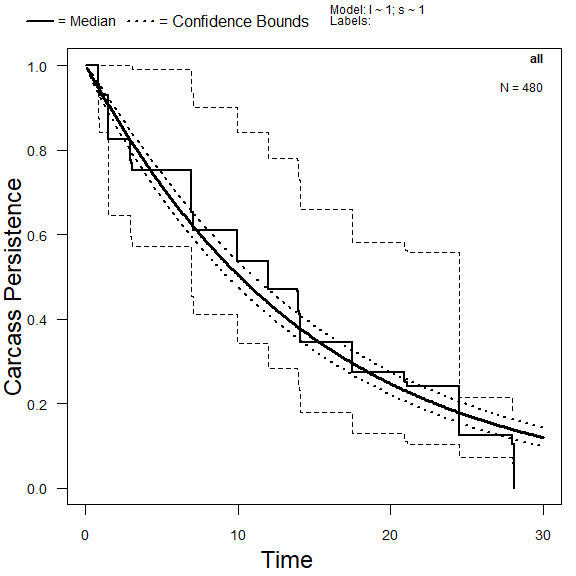to visualize the model’s output.

You can generate random draws of the $$l$$ and $$s$$ (or $$a$$ and $$b$$) parameters for each cell grouping (in cpModel there are no predictors, so there is one cell grouping called “all”) using the rcp function which, like other r* functions in R (e.g., rnorm) takes the number of random draws (n) as the first argument:

rcp(n = 10, cpModel)
#> $all #> l s #> [1,] 2.661034 0.9401477 #> [2,] 2.695537 1.0093187 #> [3,] 2.650413 0.9775857 #> [4,] 2.661567 0.9042586 #> [5,] 2.676119 0.9770536 #> [6,] 2.723761 0.9622931 #> [7,] 2.615709 0.9956862 #> [8,] 2.711809 0.9297025 #> [9,] 2.577074 0.9776859 #> [10,] 2.697527 1.0026546 rcp(n = 10, cpModel, type = "ppersist") #>$all
#>            pda      pdb
#>  [1,] 1.100073 13.23120
#>  [2,] 1.031075 16.32313
#>  [3,] 1.086564 14.76799
#>  [4,] 1.007767 14.19742
#>  [5,] 1.153752 13.95403
#>  [6,] 1.004484 14.88011
#>  [7,] 1.022291 14.11321
#>  [8,] 1.102182 13.41106
#>  [9,] 1.056327 14.11021
#> [10,] 1.040955 13.73760

You can complicate the $$l$$ and $$s$$ formulae independently

cpm(formula_l = l ~ Visibility * GroundCover, formula_s = s ~ 1, data = data_CP,
left = "LastPresentDecimalDays", right = "FirstAbsentDecimalDays",
dist = "weibull"
)
#> $call #> cpm0(formula_l = formula_l, formula_s = formula_s, data = data, #> left = left, right = right, dist = dist, CL = CL, quiet = quiet) #> #>$formula_l
#> l ~ Visibility * GroundCover
#>
#> $formula_s #> s ~ 1 #> #>$distribution
#>  "weibull"
#>
#> $predictors #>  "Visibility" "GroundCover" #> #>$AICc
#>  2109.36
#>
#> $convergence #>  0 #> #>$cell_ls
#>   cell  n l_median l_lwr l_upr s_median s_lwr s_upr
#> 1  H.A 80    2.647 2.457 2.836    0.964 0.898 1.034
#> 2  L.A 80    2.618 2.427 2.809    0.964 0.898 1.034
#> 3  M.A 80    2.536 2.350 2.722    0.964 0.898 1.034
#> 4  H.B 80    2.789 2.597 2.981    0.964 0.898 1.034
#> 5  L.B 80    2.710 2.521 2.900    0.964 0.898 1.034
#> 6  M.B 80    2.716 2.527 2.905    0.964 0.898 1.034
#>
#> $cell_ab #> cell n pda_median pda_lwr pda_upr pdb_median pdb_lwr pdb_upr #> 1 H.A 80 1.037 0.967 1.114 14.112 11.670 17.047 #> 2 L.A 80 1.037 0.967 1.114 13.708 11.325 16.593 #> 3 M.A 80 1.037 0.967 1.114 12.629 10.486 15.211 #> 4 H.B 80 1.037 0.967 1.114 16.265 13.423 19.708 #> 5 L.B 80 1.037 0.967 1.114 15.029 12.441 18.174 #> 6 M.B 80 1.037 0.967 1.114 15.120 12.516 18.265 #> #>$CL
#>  0.9
#>
#> $cell_desc #> cell medianCP r1 r3 r7 r14 r28 #> 1 H.A 9.910449 0.9691191 0.9076888 0.7965531 0.6402617 0.4355206 #> 2 L.A 9.626732 0.9681953 0.9050529 0.7912752 0.6323916 0.4266794 #> 3 M.A 8.868981 0.9654396 0.8972323 0.7757825 0.6096922 0.4019134 #> 4 H.B 11.422439 0.9732702 0.9196215 0.8208024 0.6773283 0.4789728 #> 5 L.B 10.554432 0.9710322 0.9131703 0.8076196 0.6569919 0.4547593 #> 6 M.B 10.618339 0.9712095 0.9136797 0.8086542 0.6585719 0.4566077 Given that the exponential only has one parameter ($$l$$, location), a model for scale (formula_s) is not required: cpModExp <- cpm(formula_l = l ~ Visibility * GroundCover, data = data_CP, left = "LastPresentDecimalDays", right = "FirstAbsentDecimalDays", dist = "exponential" ) ### Set of Carcass Persistence Models If the arg allCombos = TRUE is provided, cpm fits a set of cpm models defined as all allowable models simpler than, and including, the provided model formulae (where “allowable” means that any interaction terms have all component terms included in the model). In addition, cpm with allCombos can include any subset of the four base distributions (exponential, weibull, lognormal, loglogistic) and crosses them with the predictor models. Consider the following model set analysis, where Visibility and Season are included in the $$l$$ formula but only Visibility is in the $$s$$ formula, and only the exponential and lognormal distributions are included. This generates a set of 15 models: cpmModSet <- cpm(formula_l = l ~ Visibility * Season, formula_s = s ~ Visibility, data = data_CP, left = "LastPresentDecimalDays", right = "FirstAbsentDecimalDays", dist = c("exponential", "lognormal"), allCombos = TRUE ) class(cpmModSet) #>  "cpmSet" "list" names(cpmModSet) #>  "dist: exponential; l ~ Visibility * Season; NULL" #>  "dist: exponential; l ~ Visibility + Season; NULL" #>  "dist: exponential; l ~ Season; NULL" #>  "dist: exponential; l ~ Visibility; NULL" #>  "dist: exponential; l ~ 1; NULL" #>  "dist: lognormal; l ~ Visibility * Season; s ~ Visibility" #>  "dist: lognormal; l ~ Visibility + Season; s ~ Visibility" #>  "dist: lognormal; l ~ Season; s ~ Visibility" #>  "dist: lognormal; l ~ Visibility; s ~ Visibility" #>  "dist: lognormal; l ~ 1; s ~ Visibility" #>  "dist: lognormal; l ~ Visibility * Season; s ~ 1" #>  "dist: lognormal; l ~ Visibility + Season; s ~ 1" #>  "dist: lognormal; l ~ Season; s ~ 1" #>  "dist: lognormal; l ~ Visibility; s ~ 1" #>  "dist: lognormal; l ~ 1; s ~ 1" The resulting model outputs can be compared in an AICc table aicc(cpmModSet) #> Distribution Location Formula Scale Formula AICc <U+0394>AICc #> 5 exponential l ~ 1 NULL 2100.72 0.00 #> 3 exponential l ~ Season NULL 2101.08 0.36 #> 4 exponential l ~ Visibility NULL 2104.16 3.44 #> 2 exponential l ~ Visibility + Season NULL 2104.48 3.76 #> 1 exponential l ~ Visibility * Season NULL 2108.17 7.45 #> 15 lognormal l ~ 1 s ~ 1 2159.24 58.52 #> 13 lognormal l ~ Season s ~ 1 2160.78 60.06 #> 10 lognormal l ~ 1 s ~ Visibility 2162.15 61.43 #> 14 lognormal l ~ Visibility s ~ 1 2163.19 62.47 #> 8 lognormal l ~ Season s ~ Visibility 2163.67 62.95 #> 12 lognormal l ~ Visibility + Season s ~ 1 2164.74 64.02 #> 9 lognormal l ~ Visibility s ~ Visibility 2166.13 65.41 #> 11 lognormal l ~ Visibility * Season s ~ 1 2166.91 66.19 #> 7 lognormal l ~ Visibility + Season s ~ Visibility 2167.66 66.94 #> 6 lognormal l ~ Visibility * Season s ~ Visibility 2169.79 69.07 The plot function is defined for the cpmSet class, and by default, creates a new plot window on command for each sub-model. If we want to only plot a specific single (or subset) of models from the full set, we can utilize the specificModel argument: plot(cpmModSet, specificModel = "dist: lognormal; l ~ Visibility * Season; s ~ Visibility" )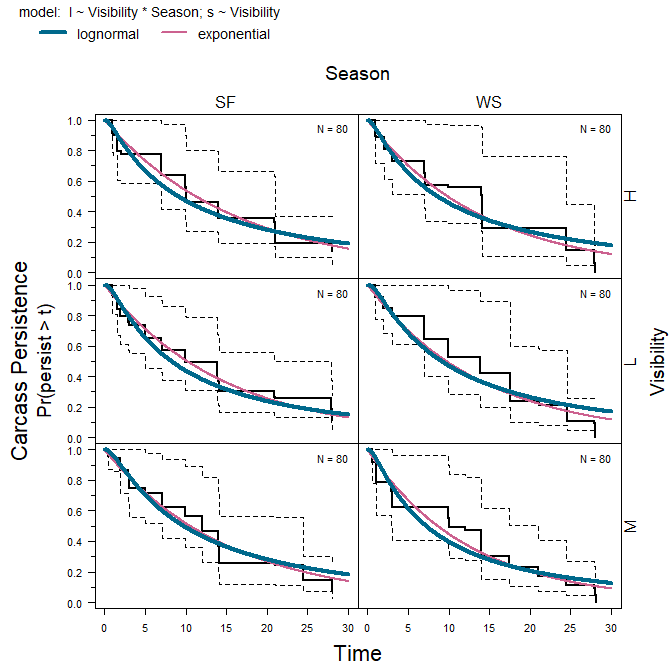### Multiple Sizes of Animals and Sets of Carcass Persistence Models Often, carcasses are grouped in multiple size classes, and we are interested in analyzing a set of models separately for each size class. To do so, we furnish cpm with sizeCol, which is the name of the column in data_CP that gives the size classes of the carcasses. If, in addition, allCombos = TRUE, then cpm returns a cpmSet for each unique size class in the column identified by the sizeCol argument: cpmModSetSize <- cpm(formula_l = l ~ Visibility * Season, formula_s = s ~ Visibility, data = data_CP, left = "LastPresentDecimalDays", right = "FirstAbsentDecimalDays", dist = c("exponential", "lognormal"), sizeCol = "Size", allCombos = TRUE) class(cpmModSetSize) #>  "cpmSetSize" "list" The cpmSetSize object is a list where each element corresponds to a different unique size class, and contains the associated cpmSeto bject, which itself is a list of cpm outputs: names(cpmModSetSize) #>  "L" "M" "S" "XL" names(cpmModSetSize[]) #>  "dist: exponential; l ~ Visibility * Season; NULL" #>  "dist: exponential; l ~ Visibility + Season; NULL" #>  "dist: exponential; l ~ Season; NULL" #>  "dist: exponential; l ~ Visibility; NULL" #>  "dist: exponential; l ~ 1; NULL" #>  "dist: lognormal; l ~ Visibility * Season; s ~ Visibility" #>  "dist: lognormal; l ~ Visibility + Season; s ~ Visibility" #>  "dist: lognormal; l ~ Season; s ~ Visibility" #>  "dist: lognormal; l ~ Visibility; s ~ Visibility" #>  "dist: lognormal; l ~ 1; s ~ Visibility" #>  "dist: lognormal; l ~ Visibility * Season; s ~ 1" #>  "dist: lognormal; l ~ Visibility + Season; s ~ 1" #>  "dist: lognormal; l ~ Season; s ~ 1" #>  "dist: lognormal; l ~ Visibility; s ~ 1" #>  "dist: lognormal; l ~ 1; s ~ 1" class(cpmModSetSize[]) #>  "cpmSet" "list" ## Generic Detection Probability For the purposes of mortality estimation, we calculate carcass-specific detection probabilities (see below), which may be difficult to generalize, given the specific history of each observed carcass. Thus, we also provide a simple means to calculate generic detection probabilities that are cell-specific, rather than carcass-specific. For any estimation of detection probability ($$\hat{g}$$), we need to have singular SE and CP models to use for each of the size classes. Here, we use the best-fit of the models for each size class: pkMods <- c("S" = "p ~ 1; k ~ 1", "L" = "p ~ 1; k ~ 1", "M" = "p ~ 1; k ~ 1", "XL" = "p ~ 1; k ~ HabitatType" ) cpMods <- c("S" = "dist: exponential; l ~ Season; NULL", "L" = "dist: exponential; l ~ 1; NULL", "M" = "dist: exponential; l ~ 1; NULL", "XL" = "dist: exponential; l ~ 1; NULL" ) The estgGenericSize function produces n random draws of generic (i.e., cell-specific, not carcass-sepecific) detection probabilities for each of the possible carcass cell combinations across the selected SE and CP models across the size classes. estgGeneric is a single-size-class version of function and estgGenericSize actually loops over estgGeneric. The generic $$\hat{g}$$ is estimated according to a particular search schedule. When we pass averageSS a full data_SS table like we have here, it will assume that columns filled exclusively with 0s and 1s represent search schedules for units and will create the average search schedule across the units. data_SS <- mock$SS
avgSS <- averageSS(data_SS)

gsGeneric <- estgGenericSize(nsim = 1000, days = avgSS,
modelSetSize_SE = pkmModSetSize,
modelSetSize_CP = cpmModSetSize,
modelSizeSelections_SE = pkMods,
modelSizeSelections_CP = cpMods
)

The output from estgGeneric can be simply summarized

summary(gsGeneric)
#> $L #> Group 5% 25% 50% 75% 95% #> 1 all 0.377 0.406 0.431 0.453 0.482 #> #>$M
#>   Group    5%   25%   50%   75%   95%
#> 1   all 0.352 0.382 0.405 0.428 0.458
#>
#> $S #> Season 5% 25% 50% 75% 95% #> 1 SF 0.358 0.396 0.425 0.451 0.489 #> 2 WS 0.300 0.333 0.358 0.383 0.420 #> #>$XL
#>   HabitatType    5%   25%   50%   75%   95%
#> 1         HT1 0.316 0.347 0.369 0.392 0.427
#> 2         HT2 0.329 0.359 0.384 0.407 0.440

or plotted.

plot(gsGeneric)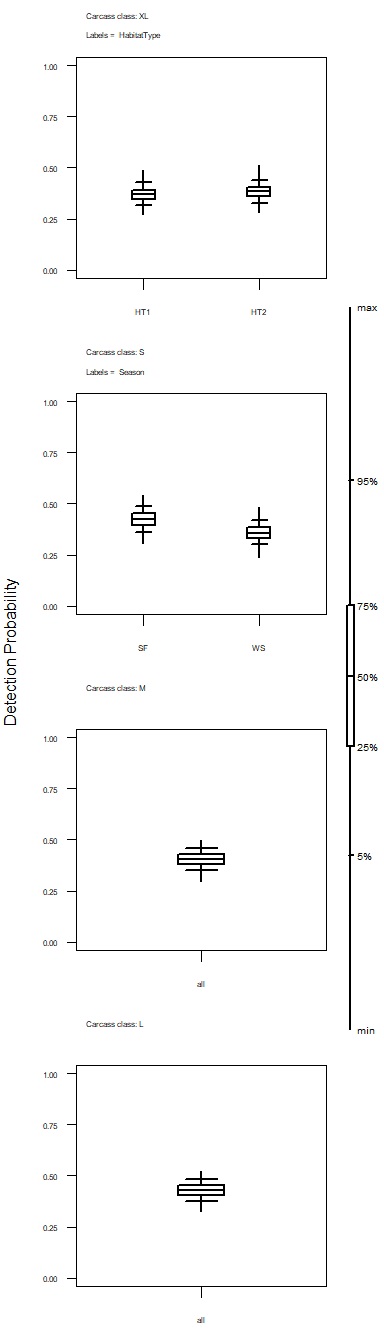## Mortality Estimation

When estimating mortality, detection probability is determined for individual carcasses based on the dates when they are observed, size class values, associated covariates, the searcher efficiency and carcass persistence models, and the search schedule. The carcass-specific detection probabilities (as opposed to the generic/cell-specific detection probabilities above) are therefore calculated before estimating the total mortality. Although it is possible to estimate these detection probabilities separately, they are best interpreted in the context of a full mortality estimation.

The estM function is the general wrapper function for estimating M, whether for a single size class or multiple size classes. Prior to estimation, we need to reduce the model-set-size complexed to just a single chosen model per size class, corresponding to the pkMods and cpMods vectors given above. To reduce the model set complexity, we can use the trimSetSize function:

pkmModSize <- trimSetSize(pkmModSetSize, pkMods)
cpmModSize <- trimSetSize(cpmModSetSize, cpMods)

In addition to the models and search schedule data, estM requires density-weighted proportion (DWP) and carcass observation (CO) data. If more than one size class is represented in the data, a required input is also the column names associated with the DWP value for each size class (argument DWPCol in estM):

data_CO <- mock$CO data_DWP <- mock$DWP
#>    Unit    S    M    L   XL
#> 1 Unit1 0.70 0.70 0.60 0.60
#> 2 Unit2 0.70 0.70 0.60 0.60
#> 3 Unit3 0.56 0.56 0.48 0.48
#> 4 Unit4 0.56 0.56 0.48 0.48
#> 5 Unit5 0.70 0.70 0.60 0.60
DWPcolnames <- names(pkmModSize)

eM <- estM(data_CO = data_CO, data_SS = data_SS, data_DWP = data_DWP,
frac = 1, model_SE = pkmModSize, model_CP = cpmModSize,
unitCol = "Unit", COdate = "DateFound",
SSdate = "DateSearched", sizeCol = "Size", nsim = 1000)

estM returns an object that contains the random draws of pkm and cpm parameters (named pk and ab, respectively) and the estimated carcass-level detection parameters (g), arrival intervals (Aj), and associated total mortality (Mhat) values for each simulation. These Mhat values should be considered in combination, and can be summarized and plotted simply:

summary(eM)
#>  median      5%     95%
#> 1799.61 1640.27 1992.19
plot(eM)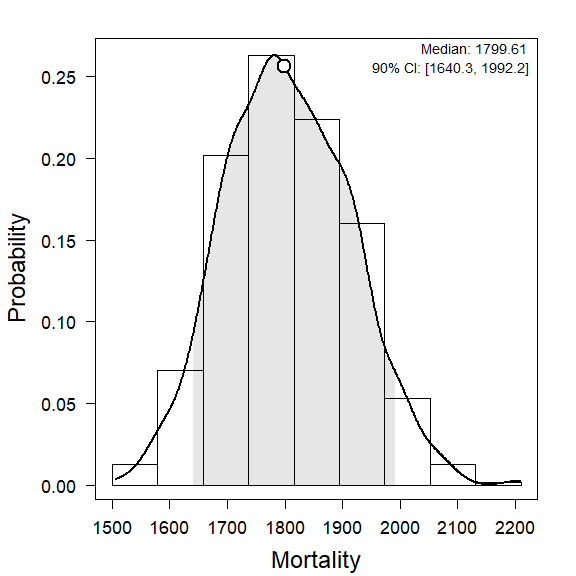### Splitting Mortality Estimations

It is possible to split the resulting mortality estimation into components that are denoted according to covariates in either the search schedule or carcass observation data sets.

First, a temporal split:

M_season <- calcSplits(M = eM, split_SS = "Construction",
split_CO = NULL, data_SS = data_SS, data_CO = data_CO
)
summary(M_season)
#>               X        5%       25%       50%       75%       95%
#> Before 161.4177  587.5406  635.8216  668.5202  709.3488  773.2055
#> After  264.5823 1006.0829 1075.6652 1133.5035 1184.7573 1266.1454
#> attr(,"CL")
#>  0.9
#> attr(,"vars")
#>  "Construction"
#> attr(,"type")
#>  "SS"
#> attr(,"times")
#>    0 127 349
#> attr(,"class")
#>  "splitSummary"
plot(M_season)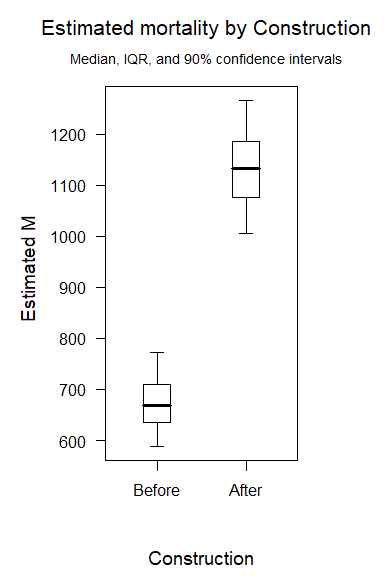Next, a carcass split:

M_class <- calcSplits(M = eM, split_SS = NULL,
split_CO = "Split", data_SS = data_SS, data_CO = data_CO
)
summary(M_class)
#>      X       5%      25%      50%       75%       95%
#> C1 196 728.3490 783.7962 823.1507  866.7523  939.2328
#> C2 230 861.2501 933.0555 976.5439 1023.1335 1109.5561
#> attr(,"CL")
#>  0.9
#> attr(,"vars")
#>  "Split"
#> attr(,"type")
#>  "CO"
#> attr(,"class")
#>  "splitSummary"
plot(M_class)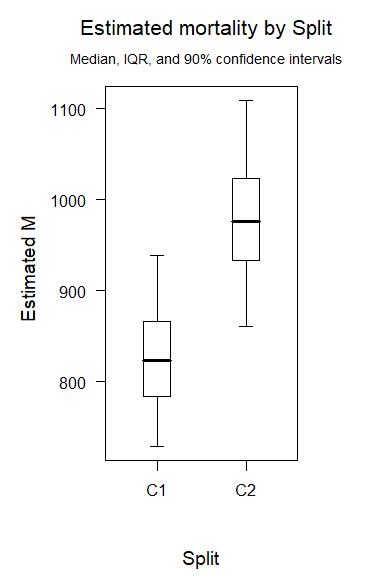And finally, if two splits are included, the mortality estimation is expanded fully factorially:

M_SbyC <- calcSplits(M = eM, split_SS = "Construction",
split_CO = "Split", data_SS = data_SS, data_CO = data_CO
)
summary(M_SbyC)
#> $C1 #> X 5% 25% 50% 75% 95% #> Before 80.19664 276.9710 306.6411 330.9697 354.7113 397.0564 #> After 115.80336 419.1385 459.5814 495.0472 524.2927 568.9108 #> #>$C2
#>                X       5%      25%      50%      75%      95%
#> Before  81.22107 279.6636 314.4793 339.1652 365.8375 408.9377
#> After  148.77893 553.2627 599.5130 637.7397 674.3206 730.7033
#>
#> attr(,"CL")
#>  0.9
#> attr(,"vars")
#>  "Construction" "Split"
#> attr(,"type")
#>  "SS" "CO"
#> attr(,"times")
#>    0 127 349
#> attr(,"class")
#>  "splitSummary"
plot(M_SbyC)